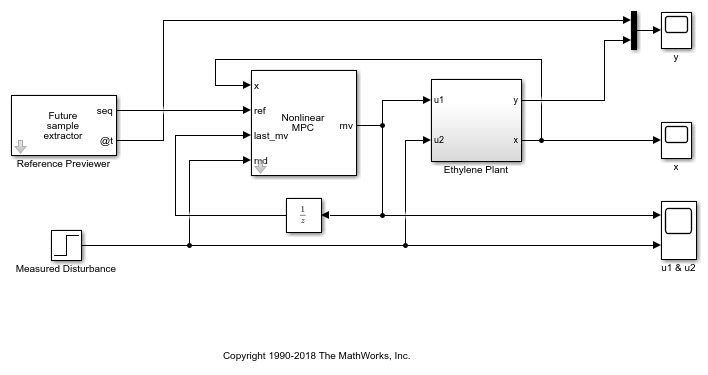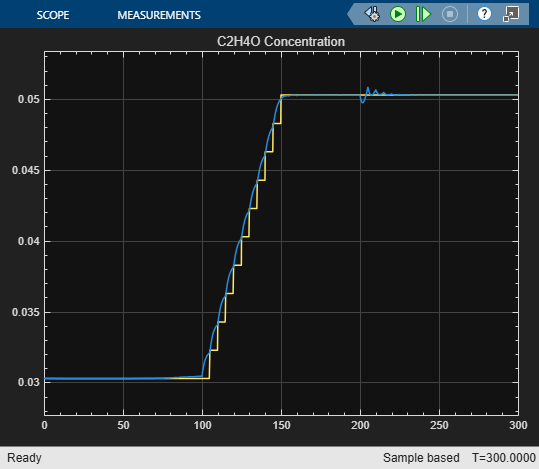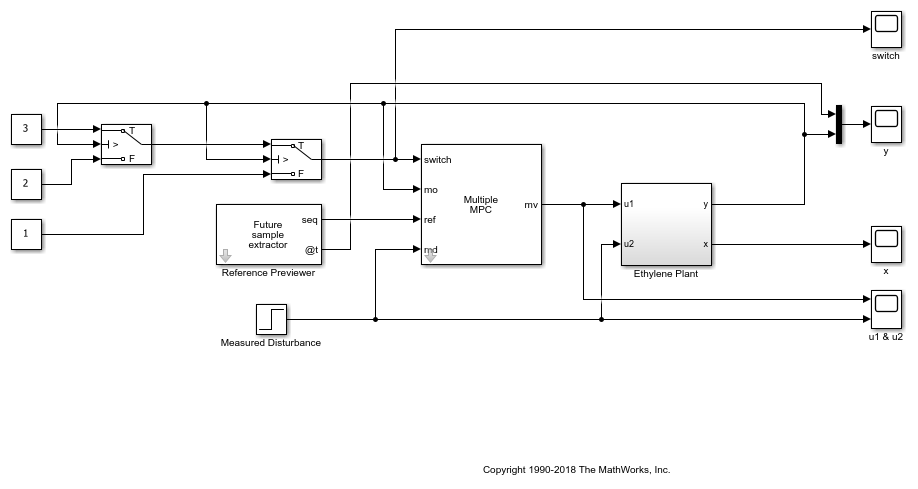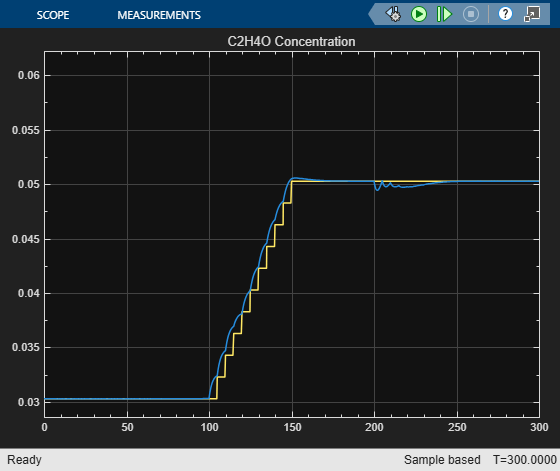Documentation

## Nonlinear and Gain-Scheduled MPC Control of an Ethylene Oxidation Plant

This example shows how to use a nonlinear model predictive controller to control an ethylene oxidation plant as it transitions from one operating point to another. In addition, linear MPC controllers are generated directly from the nonlinear MPC controller to implement a gain-scheduled control scheme that produces comparable performance.

### Product Requirement

This example requires Optimization Toolbox™ software to solve a nonlinear programming problem at each control interval.

```if ~mpcchecktoolboxinstalled('optim') disp('Optimization Toolbox is required to run this example.') return end ```

This example also requires Simulink® software to simulate MPC control of the ethylene oxidation plant in Simulink.

```if ~mpcchecktoolboxinstalled('simulink') disp('Simulink is required to run this example.') return end ```

Add example file folder to MATLAB® path.

```addpath(fullfile(matlabroot,'examples','mpc','main')); ```

### Ethylene Oxidation Plant

Oxidation of ethylene (C2H4) to ethylene oxide (C2H4O) occurs in a cooled, gas-phase catalytic reactor. Three reactions occur simultaneously in the well-mixed gas phase within the reactor:

```C2H4 + 0.5*O2 -> C2H4O ```
```C2H4 + 3*O2 -> 2*CO2 + 2*H2O ```
```C2H4O + 2.5*O2 -> 2*CO2 + 2*H2O ```

A mixture of air and ethylene is fed continuously. The plant is described by a first-principle nonlinear dynamic model, implemented as a set of ordinary differential equations (ODEs) in the `oxidationStateFcn` function. For more information, see `oxidationStateFcn.m`.

The plant contains four states:

• Gas density in the reactor (x1)

• C2H4 concentration in the reactor (x2)

• C2H4O concentration in the reactor (x3)

• Temperature in the reactor (x4)

The plant has two inputs:

• Total volumetric feed flow rate (u1)

• C2H4 concentration of the feed (u2)

The plant has one output:

• C2H4O concentration in the effluent flow (y, equivalent to x3)

For convenience, all variables in the model are pre-scaled to be dimensionless. All the states are measurable. The plant equations and parameters are obtained from .

### Control Objectives

In this example, the total volumetric feed flow rate (u1) is the manipulated variable (MV) and C2H4O concentration in the effluent flow (y) is the output variable (OV). Good tracking performance of y is required within an operating range from `0.03` to `0.05`. The corresponding u1 values are `0.38` and `0.15`, respectively.

The C2H4 concentration in the feed flow (u2) is a measured disturbance. Its nominal value is `0.5`, and a typical disturbance has a size of `0.1`. The controller is required to reject such a disturbance.

The manipulated variable u1 has a range from `0.0704` to `0.7042` due to actuator limitations.

### Feedback Control with Nonlinear MPC

In general, using nonlinear MPC with an accurate nonlinear prediction model provides a benchmark performance; that is, the best control solution you can achieve. However, in practice, linear MPC control solutions, such as adaptive MPC or gain-scheduled MPC, are more computationally efficient than nonlinear MPC. If your linear control solution can deliver a comparable performance, there is no need to implement the nonlinear control solution, especially in a real-time environment.

In this example, you first design a nonlinear MPC controller to obtain the benchmark performance. Afterward, you generate several linear MPC objects from the nonlinear controller at different operating point using the `convertToMPC` function. Finally, you implement gain-scheduled MPC using these linear MPC objects and compare the performance.

Create a nonlinear MPC controller with `4` states, `1` output, `1` manipulated variable, and `1` measured disturbance.

```nlobj = nlmpc(4,1,'MV',1,'MD',2); ```

Specify the controller sample time and the prediction and control horizons.

```Ts = 5; PredictionHorizon = 10; ControlHorizon = 3; nlobj.Ts = Ts; nlobj.PredictionHorizon = PredictionHorizon; nlobj.ControlHorizon = ControlHorizon; ```

Specify the nonlinear prediction model using `oxidationStateFcn.m`, which is a continuous-time model.

```nlobj.Model.StateFcn = 'oxidationStateFcn'; nlobj.States(1).Name = 'Den'; nlobj.States(2).Name = 'CE'; nlobj.States(3).Name = 'CEO'; nlobj.States(4).Name = 'Tc'; ```

Specify the output function that returns the C2H4O concentration in the effluent flow (same as x3). Its scale factor is its typical operating range.

```nlobj.Model.OutputFcn = @(x,u) x(3); nlobj.OV.Name = 'CEOout'; nlobj.OV.ScaleFactor = 0.03; ```

Specify the MV constraints based on the controller actuator limitations. Its scale factor is its typical operating range.

```nlobj.MV.Min = 0.0704; nlobj.MV.Max = 0.7042; nlobj.MV.Name = 'Qin'; nlobj.MV.ScaleFactor = 0.6; ```

Specify the measured disturbance name. Its scale factor is its typical operating range.

```nlobj.MD.Name = 'CEin'; nlobj.MD.ScaleFactor = 0.5; ```

Initially the plant is at an equilibrium operating point with a low concentration of C2H4O (y = `0.03`) in the effluent flow. Find the initial values of the states and output using `fsolve` from the Optimization Toolbox.

```options = optimoptions('fsolve','Display','none'); uLow = [0.38 0.5]; xLow = fsolve(@(x) oxidationStateFcn(x,uLow),[1 0.3 0.03 1],options); yLow = xLow(3); ```

Validate that the prediction model functions do not have any numerical issues using the `validateFcns` command. Validate the functions at the initial state and output values.

```validateFcns(nlobj,xLow,uLow(1),uLow(2)); ```
```Model.StateFcn is OK. Model.OutputFcn is OK. Analysis of user-provided model, cost, and constraint functions complete. ```

Specify the reference signal in a structure, where it ramps up from `0.03` to `0.05` in `50` seconds at time `100`.

```Tstop = 300; time = (0:Ts:(Tstop+PredictionHorizon*Ts))'; r = [yLow*ones(sum(time<100),1);linspace(yLow,yLow+0.02,11)';(yLow+0.02)*ones(sum(time>150),1)]; ref.time = time; ref.signals.values = r; ```

To assess nonlinear MPC performance, use a Simulink model. The Nonlinear MPC Controller block in the model is configured to use `nlobj` as its controller.

```mdlNMPC = 'oxidationNMPC'; open_system(mdlNMPC) ```Run the simulation, and view the output.

```sim(mdlNMPC) open_system([mdlNMPC '/y']) ```The nonlinear MPC controller produces good reference tracking and disturbance rejection performance, as expected.

Although a ramp-like set-point change in C2H4O concentration occurs between 100 and 150 seconds (the yellow stair curve in the plot), the controller knows about the change as early as at 50 seconds because of reference previewing. Since the objective is to minimize tracking errors across the whole horizon, the controller decides to move the plant in advance such that tracking error is the smallest across the prediction horizon. If previewing is disabled, the controller would start reacting at 100 seconds, which would produce a larger tracking error.

In this example, since all the states are measurable, full state feedback is used by the nonlinear MPC controller. In general, when there are unmeasurable states, you must design a nonlinear state estimator, such as an extended Kalman filter (EKF) or a moving horizon estimator (MHE).

### Obtain Linear MPC Controllers from Nonlinear MPC Controller

In practice, when producing comparable performance, linear MPC is always preferred over nonlinear MPC due to its higher computation efficiency. Since you designed a nonlinear MPC controller as a benchmark, you can convert it into a linear MPC controller at a specific operating point.

In this example, you generate three linear MPC controllers with C2H4O concentrations at `0.03`, `0.04`, and `0.05`, respectively. During the conversion, the nonlinear plant model is linearized at the specified operating point. All the scale factors, linear constraints, and quadratic weights defined in the nonlinear MPC object are retained. However, any custom nonlinear cost function or custom nonlinear equality or inequality constraints are discarded.

Generate a linear MPC controller at an operating point with low C2H4O conversion rate `y` = `0.03`. Specify the operating point using the corresponding state and input values, `xLow` and `uLow`, respectively.

```mpcobjLow = convertToMPC(nlobj,xLow,uLow); ```

Generate a linear MPC controller at an operating point with medium C2H4O conversion rate `y` = `0.04`.

```uMedium = [0.24 0.5]; xMedium = fsolve(@(x) oxidationStateFcn(x,uMedium),[1 0.3 0.03 1],options); mpcobjMedium = convertToMPC(nlobj,xMedium,uMedium); ```

Generate a linear MPC controller at an operating point with high C2H4O conversion rate `y` = `0.05`.

```uHigh = [0.15 0.5]; xHigh = fsolve(@(x) oxidationStateFcn(x,uHigh),[1 0.3 0.03 1],options); mpcobjHigh = convertToMPC(nlobj,xHigh,uHigh); ```

### Feedback Control with Gain-Scheduled MPC

Implement a gain-scheduled MPC solution using the three generated linear MPC controllers. The scheduling scheme is:

• If y is lower than 0.035, use `mpcobjLow`.

• If y is higher than 0.045, use `mpcobjHigh`.

• Otherwise, use `mpcobjMedium`.

To assess the gain-scheduled controller performance, use another Simulink model.

```mdlMPC = 'oxidationMPC'; open_system(mdlMPC) ```Run the simulation, and view the output.

```sim(mdlMPC) open_system([mdlMPC '/y']) ```
```-->Converting model to discrete time. -->Assuming output disturbance added to measured output channel #1 is integrated white noise. -->The "Model.Noise" property of the "mpc" object is empty. Assuming white noise on each measured output channel. -->Converting model to discrete time. -->Assuming output disturbance added to measured output channel #1 is integrated white noise. -->The "Model.Noise" property of the "mpc" object is empty. Assuming white noise on each measured output channel. -->Converting model to discrete time. -->Assuming output disturbance added to measured output channel #1 is integrated white noise. -->The "Model.Noise" property of the "mpc" object is empty. Assuming white noise on each measured output channel. ```The gain-scheduled controller produces comparable reference tracking and disturbance rejection performance.

### Conclusion

This example illustrates a general workflow to:

• Design and simulate a nonlinear MPC controller in MATLAB and Simulink for a benchmark control performance.

• Use the nonlinear MPC object to directly generate linear MPC controllers at desired operating points.

• Implement a gain-scheduled MPC control scheme using these controllers.

If the performance of the gain-scheduled controller is comparable to that of the nonlinear controller, you can feel confident implementing a linear control solution to a nonlinear control problem.

Remove example file folder from MATLAB path, and close Simulink model.

```rmpath(fullfile(matlabroot,'examples','mpc','main')); bdclose(mdlNMPC) ```

### References

 H. Durand, M. Ellis, P. D. Christofides. "Economic model predictive control designs for input rate-of-change constraint handling and guaranteed economic performance." Computers and Chemical Engineering, Vol. 92, 2016, pp. 18-36.